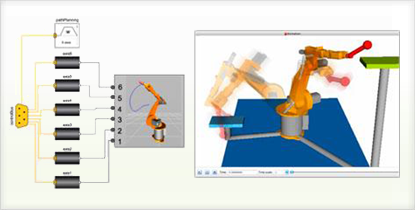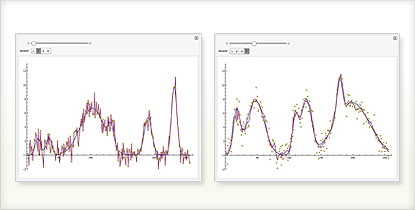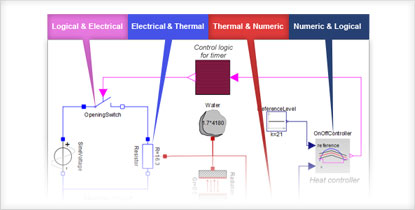# The Wolfram Solution for Electrical Engineering

Perform sophisticated image and signal processing, design and analyze control systems and create interactive models—all in one system, with one integrated workflow.

Underlying the Wolfram electrical engineering solution are advanced random processes including Markov chains and queueing processes, industrial-strength Boolean computation and high-performance dense and sparse linear algebra algorithms, all combined with the reliability of powerful symbolic and numeric computation.

• The Wolfram Edge
• How Wolfram Compares
• Key Capabilities

Wolfram technologies include thousands of built-in functions that let you:

• Apply filters and signal analysis to audio, image or other data
• Automatically create PID controllers to meet your design criteria
• Perform advanced 2D and 3D volumetric image processing
• Model electrical power distribution systems
• Design and simulate human interface devices and electronic systems with mechanical subassemblies and control units
• Analyze communication systems using continuous-time frequency domain analysis techniques
• Simulate filter circuits
• Design, analyze and simulate linear and nonlinear control systems
• Use built-in machine learning capabilitiesSimulating the path planning and control of an industrial robot with Wolfram SystemModelerDenoising a signal dynamically using the discrete wavelet transform

• Free-form linguistic input produces immediate results without the need for syntax
Unique to Wolfram technologies
• Built-in functionality for solving local and global optimization problems, both numeric and symbolic, including constrained nonlinear optimization
Matlab requires extra-cost toolboxes
• Automatic, interactive interface construction to visualize your simulations, examine model sensitivity to parameter changes and more
Unique to Wolfram technologies
• Automated precision control and arbitrary-precision numerics produce highly accurate results
Matlab and other systems that rely on finite-precision numerics can cause serious errors due to lack of precision
• Symbolic as well as numeric calculations to manipulate numbers, equations or pieces of code, improving accuracy or creating reusable models
Matlab's built-in routines only handle numeric calculations
• Highly optimized superfunctions analyze your equations and automatically select the right algorithms to get accurate results quickly—sometimes switching mid-calculation for further optimization
Other computation systems make you analyze your equations manually to determine which function to apply—for example, to solve a differential equation in Matlab, you must correctly choose among ode45, ode23, ode113, ode15s, bvp4c, pdepe and so on, or risk wrong answers
• Excellent speeds for many different types of computations, including dense and sparse linear algebra operations
Matlab is significantly slower for many of these operationsModeling the different physical domains such as electrical, thermal and logical units in an electric kettle with Wolfram SystemModelerInteractively exploring the relationship between the difference in frequencies of the waveforms and the resulting beat frequency

The Wolfram Language includes thousands of built-in functions for computation, modeling, visualization, development and deployment »

Electrical engineering specific capabilities:

Wolfram SystemModeler is the most complete physical modeling and simulation tool for high-fidelity modeling. Capabilities include:

# Consulting Solutions

Enlist the world’s computation experts to help with your project—any size, any level. At Wolfram, we know what’s possible with computational technology because we are global leaders in creating it. That gives us an unprecedented depth of expertise in applying it to consulting work in a variety of fields. Whether individual or enterprise, from concept to deployment, our computation experts can help you achieve robust results with less time and effort. Get us started with your project today